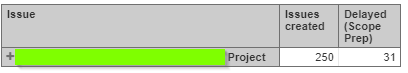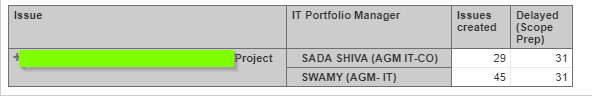cancel
Showing results for
Did you mean:
See all
See all
##### Top groups
Explore all groups

## Come for the products,stay for the community

The Atlassian Community can help you and your team get more value out of Atlassian products and practices.4,365,109
Community Members

Community Events
168
Community Groups

# Aggregation of a Calculated Measure at Dimension Level

Hi, Everyone,

I have to calculate the number of instances(issue count), there is a delay based on the planned date and the actual date.

For this purpose, I have created a calculated measure to calculate the delay.

"[Measures].[Variation(Scope Prep)]" = DateDiffWorkdays([Measures].[Issue Scope Preparation Planned End Date],  [Measures].[Issue Scope Preparation Actual End Date])

Using this calculated measure, I have created another calculated measure to get the count and get is aggregated at the dimension level

"[Measures].[Delayed (Scope Prep)]" = NonZero( Sum(
Filter(
Descendants([Issue].CurrentHierarchyMember, [Issue].[Issue]),
[Measures].[Variation(Scope Prep)]>0
),
[Measures].[Variation(Scope Prep)].Count
)
)

I have got the aggregation at the project level correctly. However, the measure is displaying the same value at each row, even if I add another dimension to the rows, which is the wrong count.Please guide me on how to get values aggregated at dimension level (similar to that of the measure Issues Created)